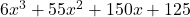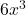The dimensions of a box are x, 2x, and 3x. Each dimension is increased by 5. Calculate the volume of the box.

Question

The dimensions of a box are x, 2x, and 3x. Each dimension is increased by 5. Calculate the volume of the box.

in progress 0
6 months 2021-07-26T01:53:21+00:00 1 Answers 3 views 0Step-by-step explanation:

the volume of the box with given dimensions is :

• x × 2x × 3x
• 6x^3

=》the measures of sides after increase in each dimension by 5 are :

1. x + 5
2. 2x + 5
3. 3x + 5

so, volume =

• (x+5) × (2x+5) × (3x+5)
• (2x^2 + 5x + 10x + 25) × (3x+5)
• (2x^2 + 15x + 25) × (3x+5)
• 6x^3 + 10x^2 + 45x^2 + 75x + 75x + 125
• 6x^3 + 55x^2 + 150x + 125

volume of box after increase of 5 in each dimension is :